# The IRT Procedure

### PROC IRT Statement

Subsections:
• PROC IRT <options>;

The PROC IRT statement invokes the IRT procedure. Table 65.1 summarizes the options available in the PROC IRT statement. The sections that follow the table describe the PROC IRT statement options and then describe the other statements in alphabetical order.

Table 65.1: PROC IRT Statement Options

Option

Description

Basic Options

Specifies the input data set

Reverses the sort order of the levels of the response variable

Inputs the model specifications

Computes the item fit statistics and displays them in a table

Computes the classical item statistics and displays them in a table

Specifies the link function

Specifies the number of factors

Specifies the output data set for factor scores

Outputs the model specifications

Specifies the response function

Specifies the sort order of the response variables

Specifies the factor score estimation method

Computational Options

Specifies an absolute function difference convergence criterion

Specifies an absolute gradient convergence criterion

Specifies a maximum absolute parameter difference convergence criterion

Specifies a relative function convergence criterion

Specifies a relative gradient convergence criterion

Specifies the maximum number of function calls in the optimization process

Specifies the maximum number of iterations in the optimization process

Specifies the maximum number of iterations in the maximization step of the EM algorithm

Specifies the number of quadrature points per dimension

Specifies the optimization technique to obtain maximum likelihood estimates

Display Options

Suppresses the display of the "Iteration History" table

Suppresses all ODS output

Displays initial parameter estimates

Displays the polychoric correlation matrix

Controls plots that are produced through ODS Graphics

Rotation Method and Properties

Specifies the convergence criterion for rotation cycles

Specifies the maximum number of rotation cycles

Specifies the rotation method

#### PROC IRT Statement Options

ABSFCONV=r
ABSFTOL=r

specifies an absolute function difference convergence criterion. Termination requires a small change of the function value in successive iterations,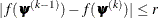where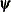denotes the vector of parameters that participate in the optimization and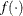is the objective function. This criterion is not used by the expectation-maximization (EM) algorithm. By default, r = 0.

ABSGCONV=r
ABSGTOL=r

specifies an absolute gradient convergence criterion. Termination requires the maximum absolute gradient element to be small,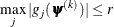wheredenotes the vector of parameters that participate in the optimization and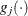is the gradient of the objective function with respect to the jth parameter. This criterion is not used by the EM algorithm. By default, r = 1E–5.

ABSPCONV=r
ABSPTOL=r

specifies a maximum absolute parameter difference convergence criterion. This criterion is used only by the EM algorithm. Termination requires the maximum absolute parameter change in successive iterations to be small,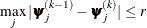where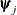denotes the jth parameter that participates in the optimization. By default, r = 1E–4.

DATA=SAS-data-set

specifies the SAS-data-set to be read by PROC IRT. The default value is the most recently created data set.

DESCENDING
DESC

reverses the sorting order for the levels of the response variables. If you specify both the DESCENDING and RORDER= options, PROC IRT orders the levels according to the RORDER= option and then reverses that order.

FCONV=r
FTOL=r

specifies a relative function convergence criterion. Termination requires a small relative change of the function value in successive iterations,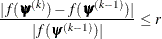wheredenotes the vector of parameters that participate in the optimization andis the objective function. This criterion is not used by the EM algorithm. By default, r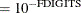, where FDIGITS is, by default,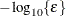andis the machine precision.

GCONV=r
GTOL=r

specifies a relative gradient convergence criterion. For all techniques except CONGRA, termination requires the normalized predicted function reduction to be small,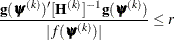wheredenotes the vector of parameters that participate in the optimization,is the objective function, and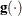is the gradient. For the CONGRA technique (for which a reliable Hessian estimateis not available), the following criterion is used: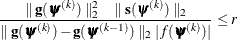This criterion is not used by the EM algorithm. By default, r = 1E–8.

INMODEL<(SCORE)>=SAS-data-set

specifies an input data set that contains information about the analysis model. Instead of specifying and running the model in a new run, you can use the INMODEL= option to input the model specification saved as an OUTMODEL= data set in a previous PROC IRT run.

Sometimes, you might want to create an INMODEL= data set by modifying an existing OUTMODEL= data set. However, editing and modifying OUTMODEL= data sets requires a good understanding of the formats and contents of the OUTMODEL= data sets. This process could be difficult for novice users. For more information about the format of INMODEL= and OUTMODEL= data sets, see the sectionOutput Data Sets.

When you specify the INMODEL= option, the VAR, MODEL, GROUP, FACTOR, VARIANCE, COV, and EQUALITY statements are ignored. The DESCENDING, LINK, NFACTOR, RESFUNC, and RORDER options in the PROC IRT statement are also ignored. When there are duplicated specifications, the first specification is used.

Specify the SCORE suboption if you want to use the model specifications and parameter estimates from the INMODEL= data set to score a new subject without refitting the model.

You can use the INMODEL= option along with the SCORE suboption for many different purposes, including the following:

• If you specify the INMODEL= option, PROC IRT fits an IRT model to the DATA= data set based on the model specifications in the INMODEL= data set and uses the parameter estimates in the INMODEL= data set as initial values.

• If you specify the INMODEL= option and the OUT= option, PROC IRT fits an IRT model to the DATA= data set based on the model specifications in the INMODEL= data set and uses the parameter estimates in the INMODEL= data set as initial values. Then PROC IRT scores the DATA= data set by using the new parameter estimates obtained in the previous step.

• If you specify the INMODEL(SCORE)= option and the OUT= option, PROC IRT scores the DATA= data set by using the model specifications and parameter estimates in the INMODEL= data set without refitting the model.

ITEMFIT

displays the item fit statistics. These item fit statistics apply only to binary items that have one latent factor.

ITEMSTAT <(itemstat-options )>

displays the classical item statistics, which include the item means, item-total correlations, adjusted item-total correlations, and item means for i ordered groups of observations or individuals. You can specify the following itemstat-options:

NPARTITION=i

specifies the number of groups, where i must be an integer between 2 and 5, inclusive. By default NPARTITION=4.

The i ordered groups are formed by partitioning subjects based on the rank of their sum scores. By default, there are four groups, labeled G1, G2, G3, and G4, representing four ascending ranges of sum scores. The formula for calculating group values is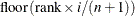where floor is the floor function, rank is the sum score’s order rank, i is the value of the NPARTITION= option, and n is the number of observations that have nonmissing values of sum scores for TIES=LOW, TIES=MEAN, and TIES=HIGH. For TIES=DENSE, n is the number of observations that have unique nonmissing sum scores. If the number of observations is evenly divisible by the number of groups, each group has the same number of observations, provided that there are no tied sum scores at the boundaries of the groups. Sum scores with many tied values can create unbalanced groups because observations that have the same sum scores are assigned to the same group.

TIES=HIGH | LOW | MEAN | DENSE

specifies how to compute normal scores or ranks for tied data values.

HIGH

assigns the largest of the corresponding ranks.

LOW

assigns the smallest of the corresponding ranks.

MEAN

assigns the mean of the corresponding rank.

DENSE

computes scores and ranks by treating tied values as a single-order statistic. For the default method, ranks are consecutive integers that begin with the number 1 and end with the number of unique, nonmissing values of the variable that is being ranked. Tied values are assigned the same rank.

By default, TIES=MEAN.

Observations (subjects) that have missing values are excluded from the computations of the classical item statistics.

specifies the link function. You can specify the following names:

LOGIT

requests the logistic link function.

PROBIT

requests the probit link function.

MAXFUNC=n
MAXFU=n

specifies the maximum number of function calls in the optimization process. This option is not used by the EM algorithm. The default values are as follows, depending on which optimization technique is specified in the TECHNIQUE= option:

• NRRIDG: 125

• QUANEW: 500

• CONGRA: 1000

The optimization can terminate only after completing a full iteration. Therefore, the number of function calls that are actually performed can exceed the number that this option specifies.

MAXITER=n
MAXIT=n

specifies the maximum number of iterations in the optimization process. The default values are as follows, depending on which optimization technique is specified in the TECHNIQUE= option:

• NRRIDG: 50

• QUANEW: 200

• CONGRA: 400

• EM: 500

MAXMITER=n
MAXMIT=n

specifies the maximum number of iterations in the maximization step of the EM algorithm. By default, MAXMITER=1.

NFACTOR=i
NFACT=i

specifies the number of factors, i, in the model. You must specify the number of factors only for exploratory analysis, in which all the slope parameters of the items are freely estimated without being explicitly constrained by using the FACTOR statement. By default, NFACTOR=1. When you use the FACTOR statement to specify the confirmatory factor pattern, the number of factors is implicitly defined by the number of distinctive factor names that you specify in the statement.

requests that the Gaussian quadrature be nonadaptive.

NOITPRINT

suppresses the display of the "Iteration History" table.

NOPRINT

suppresses all output displays.

OUT=SAS-data-set

creates an output data set that contains all the data in the DATA= data set plus estimated factor scores. For exploratory analysis, the factor scores are named _Factor1, _Factor2, and so on. For confirmatory analysis, user-specified factor names are used.

PROC IRT provides three estimation methods for factor scores. You can specify a method by using the SCOREMETHOD option. The default estimation method, maximum a posteriori (MAP), is used if the SCOREMETHOD option is not specified.

OUTMODEL=SAS-data-set

creates an output data set that contains the model specification, the parameter estimates, and their standard errors. You can use an OUTMODEL= data set as an input INMODEL= data set in a subsequent analysis by PROC IRT.

If you want to create a SAS data set in a permanent library, you must specify a two-level name. For more information about permanent libraries and SAS data sets, see SAS Language Reference: Concepts.

PINITIAL

displays the initial parameter estimates.

PLOTS <(global-plot-options)> <= plot-request <(options)>>
PLOTS <(global-plot-options)> <= (plot-request <(options)> <…plot-request <(options)>>)>

controls the plots that are produced through ODS Graphics. When you specify only one plot-request, you can omit the parentheses around it. For example:

plots=all
plots=ICC(unpack)
plots(unpack)=(scree ICC)


ODS Graphics must be enabled before plots can be requested. For example:

ods graphics on;
proc irt plots=all;
run;
ods graphics off;


For more information about enabling and disabling ODS Graphics, see the section Enabling and Disabling ODS Graphics in Chapter 21: Statistical Graphics Using ODS.

You can specify the following global-plot-options, which apply to all plots that the IRT procedure generates:

UNPACK |UNPACKPANEL

suppresses paneling. By default, multiple plots can appear in some output panels. Specify UNPACK to display each plot individually. You can also specify UNPACK as a suboption in the ICC, IIC, and SCREE options.

XVIEWMAX

specifies a maximum value for the X axis. You can also specify XVIEWMAX as a suboption in the ICC, IIC, and TIC options.

XVIEWMIN

specifies a minimum value for the X axis. You can also specify XVIEWMIN as a suboption in the ICC, IIC, and TIC options.

You can specify the following plot-requests:

ALL

displays all default plots.

ICC <(UNPACK |UNPACKPANEL), (XVIEWMAX=), (XVIEWMIN=)>

displays item characteristic curves (ICCs). By default, multiple ICC plots appear in some output panels. You can request an individual ICC plot for each item by specifying the UNPACK suboption. For binary items, the ICC plot includes only the curve for the higher category, which is often the correct response category or the endorsed category. For ordinal items that have more than two categories, the ICC plot includes curves for all the categories and also a legend with the values 0, 1, 2, and so on to indicate the curves for different categories.

IIC <(UNPACK |UNPACKPANEL), (XVIEWMAX=), (XVIEWMIN=)>

displays item information curves (IICs). By default, multiple IIC plots appear in some output panels. You can request an individual IIC plot for each item by specifying the UNPACK suboption.

NONE

suppresses all plots.

POLYCHORIC <options>
PLCORR<options>

displays a heat map of the polychoric correlation matrix. You can specify one or both of the following options:

FUZZ=p

displays polychoric correlations whose absolute values are less than p as 0 in the heat map. This option is useful when you want to focus on the patterns of sizable correlations that are larger than p in the heat map. By default, FUZZ=0.

OUTLINE=ON | OFF

specifies whether to display an outline of the regions in the polychoric correlation heat map. By default, OUTLINE=ON.

SCREE <(UNPACK |UNPACKPANEL)>

displays the scree and variance-explained plots in the same panel. You can display these plots individually by specifying the UNPACK suboption.

TIC <(XVIEWMAX=), (XVIEWMIN=)>

displays a test information curve (TIC) plot.

POLYCHORIC

displays the polychoric correlation matrix.

QPOINTS=i

specifies the number of quadrature points in each dimension of the integral. If there are d latent factors and n quadrature points, the IRT procedure evaluates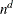conditional log likelihoods for each observation to compute one value of the objective function. Increasing the number of quadrature nodes can substantially increase the computational burden. If you do not specify the number of quadrature points, it is determined adaptively by using the initial parameter estimates.

RCONVERGE=p
RCONV=p

specifies the convergence criterion for rotation cycles. Rotation stops when the scaled change of the simplicity function value is less than the RCONVERGE= value. The default convergence criterion is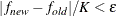where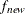and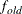are simplicity function values of the current cycle and the previous cycle, respectively;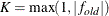is a scaling factor; andis 1E–9 by default and is modified by the RCONVERGE= value.

RESFUNC=ONEP | TWOP | THREEP | FOURP | GRADED | RASCH

specifies the response functions for the variables that are included in the VAR statement. The response functions correspond to different response models. You can specify the following values:

ONEP

specifies the one-parameter model.

TWOP

specifies the two-parameter model.

THREEP

specifies the three-parameter model.

FOURP

specifies the four-parameter model.

specifies the graded response model.

RASCH

specifies the Rasch model.

By default, RESFUNC=TWOP for binary items and RESFUNC=GRADED for ordinal items. The graded response model assumes that the response variables are ordinal-categorical up to 11 levels. All other models assume binary responses. For more information about these response models, see "Response Models" in the Details: IRT Procedure section.

RITER=n

specifies the maximum number of cycles for factor rotation. The default value is the maximum between 10 times the number of variables and 100.

RORDER=DATA | FORMATTED | FREQ | INTERNAL

specifies the sort order for the levels of the response variable. This order determines which threshold parameter in the model corresponds to each level in the data. If RORDER=FORMATTED for numeric variables for which you have supplied no explicit format, the levels are ordered by their internal values. This option applies to all the responses in the model. When the default, RORDER=FORMATTED, is in effect for numeric variables for which you have supplied no explicit format, the levels are ordered by their internal values. You can specify the following sort orders:

 Value of RORDER= Levels Sorted By DATA Order of appearance in the input data set FORMATTED External formatted value, except for numeric variables that have no explicit format, which are sorted by their unformatted (internal) value FREQ Descending frequency count; levels that contain the most observations come first in the order INTERNAL Unformatted value

For FORMATTED and INTERNAL, the sort order is machine-dependent. For more information about sort order, see the chapter on the SORT procedure in the SAS Procedures Guide and the discussion of BY-group processing in SAS Language Reference: Concepts.

ROTATE=name
R=name

specifies the rotation method.

You can specify the following orthogonal rotation methods:

BIQUARTIMAX | BIQMAX

specifies orthogonal biquartimax rotation.

EQUAMAX | E

specifies orthogonal equamax rotation.

NONE | N

specifies that no rotation be performed, leaving the original orthogonal solution.

PARSIMAX | PA

specifies orthogonal parsimax rotation.

QUARTIMAX | QMAX | Q

specifies orthogonal quartimax rotation.

VARIMAX | V

specifies orthogonal varimax rotation.

You can specify the following oblique rotation methods:

BIQUARTIMIN | BIQMIN

specifies biquartimin rotation.

COVARIMIN | CVMIN

specifies covarimin rotation.

OBBIQUARTIMAX | OBIQMAX

specifies oblique biquartimax rotation.

OBEQUAMAX | OE

specifies oblique equamax rotation.

OBPARSIMAX | OPA

specifies oblique parsimax rotation.

OBQUARTIMAX | OQMAX

specifies oblique quartimax rotation.

OBVARIMAX | OV

specifies oblique varimax rotation.

QUARTIMIN | QMIN

specifies quartimin rotation.

By default, ROTATE=VARIMAX.

SCOREMETHOD=ML | EAP | MAP

specifies the method of factor score estimation. You can specify the following methods:

ML

requests the maximum likelihood method.

EAP

requests the expected a posteriori method.

MAP

requests the maximum a posteriori method.

By default, SCOREMETHOD=MAP.

TECHNIQUE=CONGRA | EM | NONE | NRRIDG | QUANEW
TECH=CONGRA | EM | NONE | NRRIDG | QUANEW
OMETHOD=CONGRA | EM | NONE | NRRIDG | QUANEW

specifies the optimization technique to obtain maximum likelihood estimates. You can specify the following techniques:

CONGRA

performs a conjugate-gradient optimization.

EM

performs an EM optimization.

NONE

performs no optimization.

NRRIDG

performs a Newton-Raphson optimization with ridging.

QUANEW

performs a dual quasi-Newton optimization.

By default, TECHNIQUE=QUANEW.

For more information about these optimization methods (except EM), see the section Choosing an Optimization Algorithm in Chapter 19: Shared Concepts and Topics. For more information about the EM algorithm, see "Expectation-Maximization (EM) Algorithm" in the section Details: IRT Procedure.